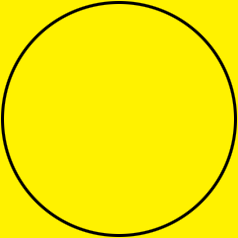SEARCH HOMEMath Central Quandaries & QueriesQuestion from Sabra: Hello, I have twins in the 7th grade that are learning how to find circumference of circles. I am struggling with figuring out how to help my children to find the answer to this question: Tye has a square piece of yellow felt that has an area of 81 square inches. She wants to cut the largest circle possible from the material to create a sun for her art project. What is the area of the felt circle? Use 3.14 for pi. Round to the nearest hundredth if necessary. I wasn't sure if I am to find the area of the circle through finding the area of the square or what steps we needed to follow to find the answer. Once I can see how one is worked out step-by-step, I usually can help them along with questions that are similar.Hi,

The area $A$ of a circle is given by

$A = \pi\; r^2$

where $r$ is the radius of the circle and you are told to approximate $\pi$ by 3.14. Similarly the circumference $C$ of a circle is given by

$C = 2 \pi\; r.$

Hence to determine the area and circumference of a circle you need to find its radius $r.$

The square piece of felt that Tye needs to cut has an area of 81 square inches. The area of a square is the square of a side length, and since $9\times 9 = 9^2 = 81,$ his square piece of felt has a side length if 9 inches.

Here is my diagram of Tye's square of felt with the largest circle possible drawn on it.What is the radius of the circle?

Write back if you need more assistance,
PennyMath Central is supported by the University of Regina and The Pacific Institute for the Mathematical Sciences.